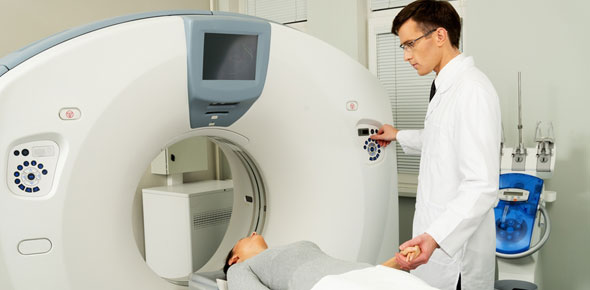# Cat Scan Review

91 Questions | Total Attempts: 223SettingsQuestion for Computed Tomography Registry

Questions and Answers
• 1.
What is an advantage of scans of the pituitary taken in the coronal plane?
• A.

IV contrast material is better visualized

• B.

Streak artifacts are reduced or eliminated.

• C.

The patient is more comfortable during scanning.

• D.

The patient receives a lower radiation dose

• 2.
Which two strategies can reduce the effect of dental fillings in a sinus study?
• A.

High mA and shcrt scan time

• B.

Low kVp and high contrast, or bone alogrithm

• C.

Adjust the angle of the gantry and widen the window width

• D.

Small focal spot and small display field

• 3.
Which of the following conditions may be visualized in a CT study of the brain?
• A.

Glioblastoma

• B.

Wilm's Tumor

• C.

Krukenberg Tumor

• D.

Plasmacytosis

• 4.
Which of the following is affected by teh slice spacing in a CT study?
• A.

Spatial Resolution

• B.

Quantum Mottle

• C.

Radiation Dose to the Patient

• D.

Signal-to-Noise Ration

• 5.
What is the name of the pinecon-shaped structure of the brain the can often be seen as calcified in CT images?
• A.

Choroid Plexus

• B.

Pineal Gland

• C.

Caudate Nucleus

• D.

Middle cerebral Artery

• 6.
Third generation (rotate/rotate) scanners overcame which of the following limitations?
• A.

Detector singal drift sensitivity

• B.

Translation motion

• C.

Spurious signals from moving signal wires

• D.

Cost

• 7.
What helical or spiral computed tomography term is defined as the table increment in distance per mm per 360 degrees gantry rotation divided by the section thickness in mm?
• A.

Rotation

• B.

Spiral

• C.

Helix

• D.

Pitch

• 8.
What determines the section thickness?
• A.

Scan arc

• B.

Filtration algorithm

• C.

Incident beam collimation

• D.

Detector alignment

• 9.
What effect would an array processor failure have on image processing?
• A.

Increase image display time

• B.

Halt analog to digital conversions

• C.

Lengthens detector data acquisition time

• D.

Decrease xray tube output

• 10.
What change will occur in the number of detected photons if a section thickness is changed from 5 mm to 10mm?
• A.

Decrease 50%

• B.

Decrease 25%

• C.

Increase 50%

• D.

Increase 100%

• 11.
Which of the following will magnify the image?
• A.

Increase field of view

• B.

Decreasing field of view

• C.

Increasing section thickness

• D.

Decreasing section thickness

• 12.
Which of the following is convolution?
• A.

Filtered back projection

• B.

Fourier transform

• C.

Iterative filtration

• D.

3-d rendering

• 13.
Which of the following determines the maximum number of shades of gray that can be displayed on the CT monitors?
• A.

Window level

• B.

Window width

• C.

Pixel size

• D.

Matrix size

• 14.
Which of the following are benefits of narrower section thicknesses?
• A.

Reduced partial volume averaging and improved spatial resolution

• B.

Decreased scan time, and more anatomy covered

• 15.
Which of the following is the term used to describe a voxel value that is the average of multiple tissue types contained within the voxel?
• A.

Linear attenuation coefficient

• B.

Partial volume averaging

• C.

Low contrast resolution

• D.

Edge gradient averaging

• 16.
Which of the following CT numbers would appear as a gray shades when a window width of 300 and a window level of 0 are selected?
• A.

Ct numbers above +300

• B.

Ct numbers between +150 and +300

• C.

Ct numbers between +150 and -150

• D.

Ct numbers below -150

• 17.
Which fo the following is the central value in a range of gray shades used to display the image?
• A.

Window width

• B.

Window level

• C.

Region of interest

• D.

Dose index

• 18.
What is the most common type of film used to recore CT exams?
• A.

Single emulsion

• B.

Double emulsion

• C.

Triple emulsion

• D.

Rare earth

• 19.
Which of the following increase signal to noise ration?
• A.

Increased mAs

• B.

Wider section thickness

• C.

Increased detection efficiency

• 20.
What is the appropriate compensation to avoid quantum noise when performing thin section computed tomography?
• A.

Increase mAs

• B.

Decrease field of view

• C.

Increase matrix size

• D.

Decrease scan speed

• 21.
Which describes the relationship between section thickness and noise?
• A.

If section thickness increases, noise increases

• B.

If section thickness increases, noise decreases

• C.

If section thickness decreases, noise decreases

• D.

If section thickness decreases, noise remains unchanged

• 22.
What term is used to describe as the ability to display an image of a large object that is only slightly different in density from its surroundings?
• A.

Spatial resolution

• B.

Contrast resolution

• C.

Linearity

• D.

Clarity

• 23.
Which of the following should be viewed with relatively wide window widths?
• A.

Lung and inner ear

• B.

Lung and brain

• C.

Inner ear and brain

• 24.
How often should an average CT number water calibration test be performed?
• A.

Daily

• B.

Monthly

• C.

Yearly

• D.

Weekly

• 25.
Which of the following accurately describes the relationship between patient dose and pitch?
• A.

If pitch increases, patient dose increases

• B.

If pitch increases, patient dose decreases

• C.

If pitch decreases, patient dose decreases

• D.

If pitch decreases, patient dose remains unchanged

Related Topics